Finding square root by division method - Decimals

Chapter 5 Class 8 Squares and Square Roots
Concept wise

Let’s do this by example

### Square root of 17.64 by long division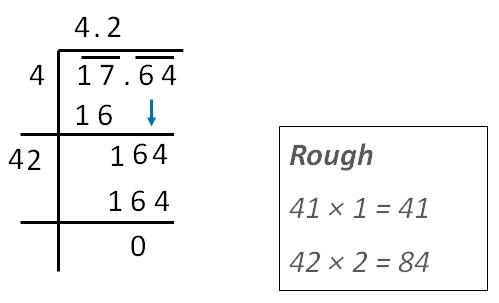Therefore,

Square root of 17.64 = 4.2

### Individual Steps are explained

Step 1:

• Write the number
• We make pairs from right.

For numbers before decimal point,

we start from right and make pairs

So, 17 is a pair

For numbers after decimal point,

we start from the right of decimal point and make pairs

So, 64 is a pair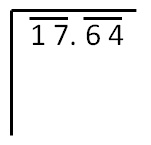Step 2:

Now we find that number whose

square is less than or equal to 16.

3 × 3 = 9

4 × 4 = 16

5 × 5 = 25

Since 25 > 17, we use 4 × 4.

So writing 4 on left side & top.

& 16 below 17

Now we find remainder,

17 − 16 = 1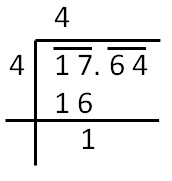Step 3:

Since next is decimal point

We put a decimal point on topStep 4

Pair 64 comes down

So, our number is now 164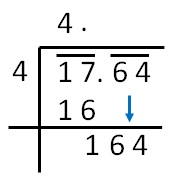Step 5

We multiply the number above by 2

& add a blank after it

So, we write 4 × 2 = 8

8 _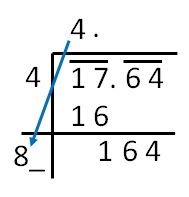Step 6

Find a number which is less than

Or equal to 164

81 × 1 = 81

82 × 2 = 164

Since 82 × 2 = 164. We use that

Thus,

Square root of 17.64 = 4.2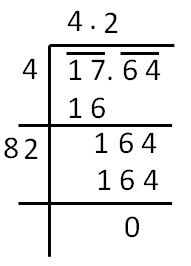### Square root of 1.125 by long division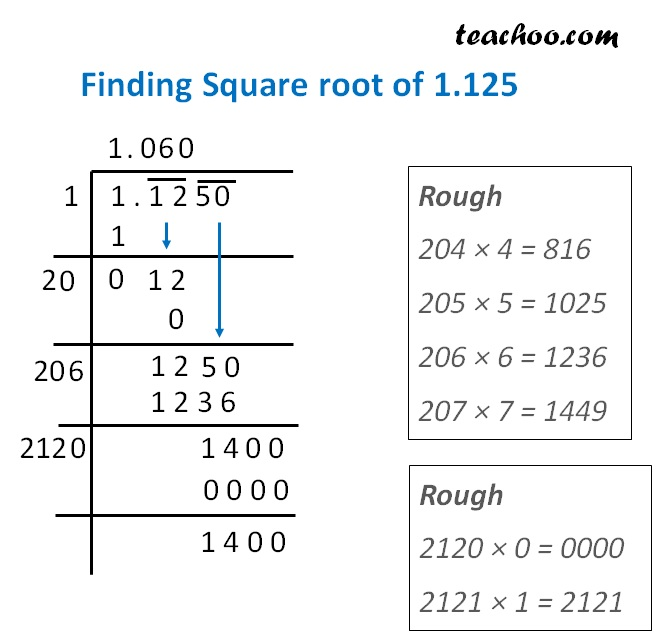Therefore,

Square root of 1.125 = 1.060…

Here, we can find square root upto more decimal digits.

But, we stop at 3 digits after decimal point

Learn in your speed, with individual attention - Teachoo Maths 1-on-1 Class